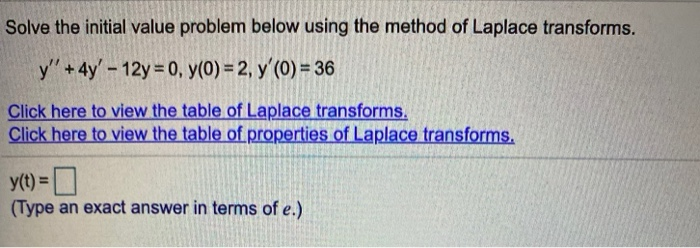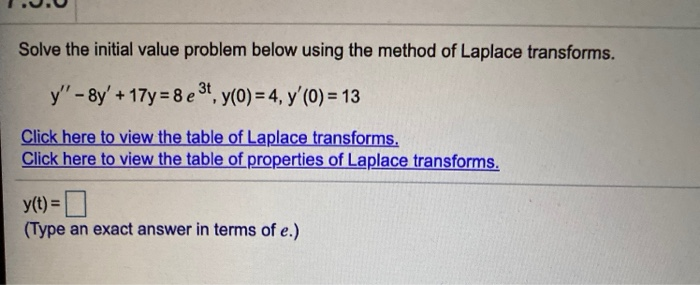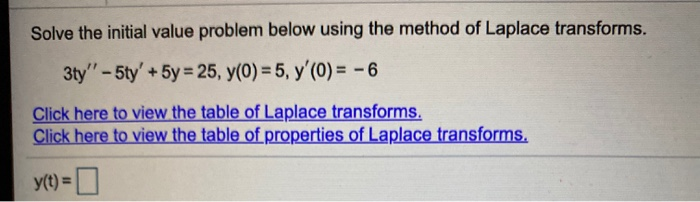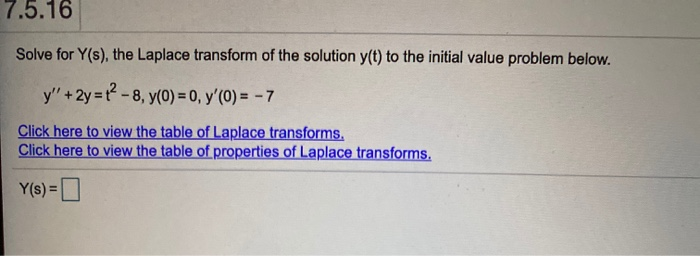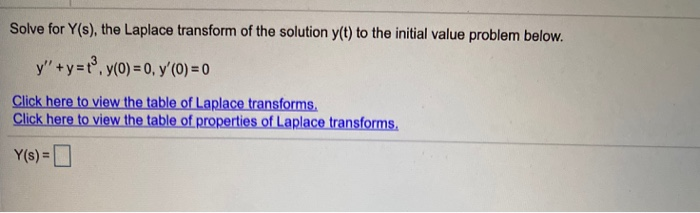Solve the initial value problem below using the method of Laplace transforms. y'' + 4y' - 12y = 0, y(0) = 2, y' (O) = 36 Click here to view the table of Laplace transforms. Click here to view the table of properties of Laplace transforms. y(t) = 0 (Type an exact answer in terms of e.)
Solve the initial value problem below using the method of Laplace transforms. y'' - 8y' + 17y = 8 e 31 y(0) = 4, y'(0) = 13 Click here to view the table of Laplace transforms. Click here to view the table of properties of Laplace transforms. y(t) = (Type an exact answer in terms of e.)
Solve the initial value problem below using the method of Laplace transforms. 3ty'' -5ty' + 5y = 25, y(0) = 5, y'(0) = -6 Click here to view the table of Laplace transforms. Click here to view the table of properties of Laplace transforms. y(t) =
7.5.16 Solve for Y(s), the Laplace transform of the solution y(t) to the initial value problem below. y" + 2y = -8, y(0) = 0, y'(0) = -7 Click here to view the table of Laplace transforms. Click here to view the table of properties of Laplace transforms. Y(s)=0
Solve for Y(s), the Laplace transform of the solution y(t) to the initial value problem below. y+y=t, y(0) = 0, y'(0) = 0 Click here to view the table of Laplace transforms. Click here to view the table of properties of Laplace transforms. Y(s) =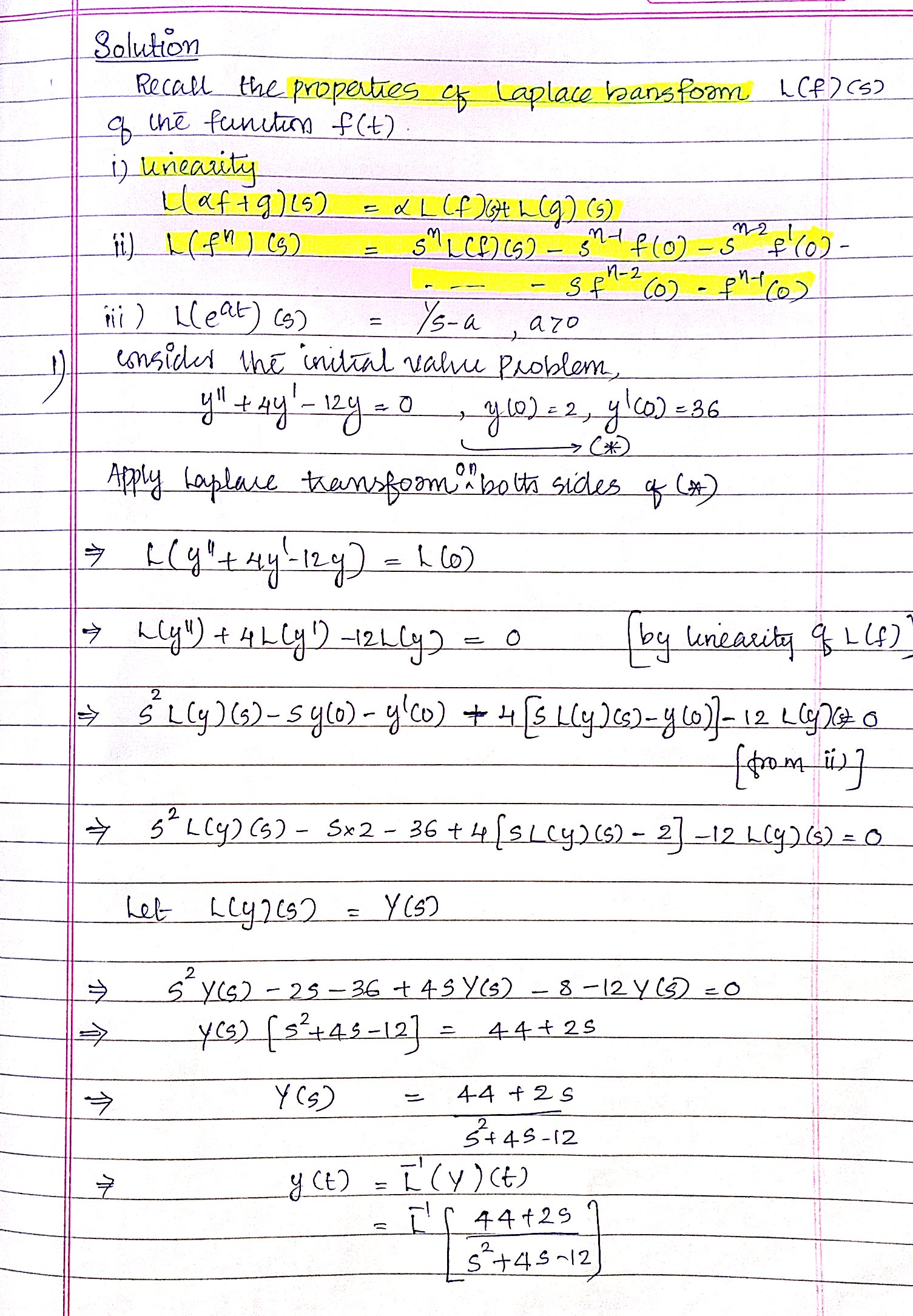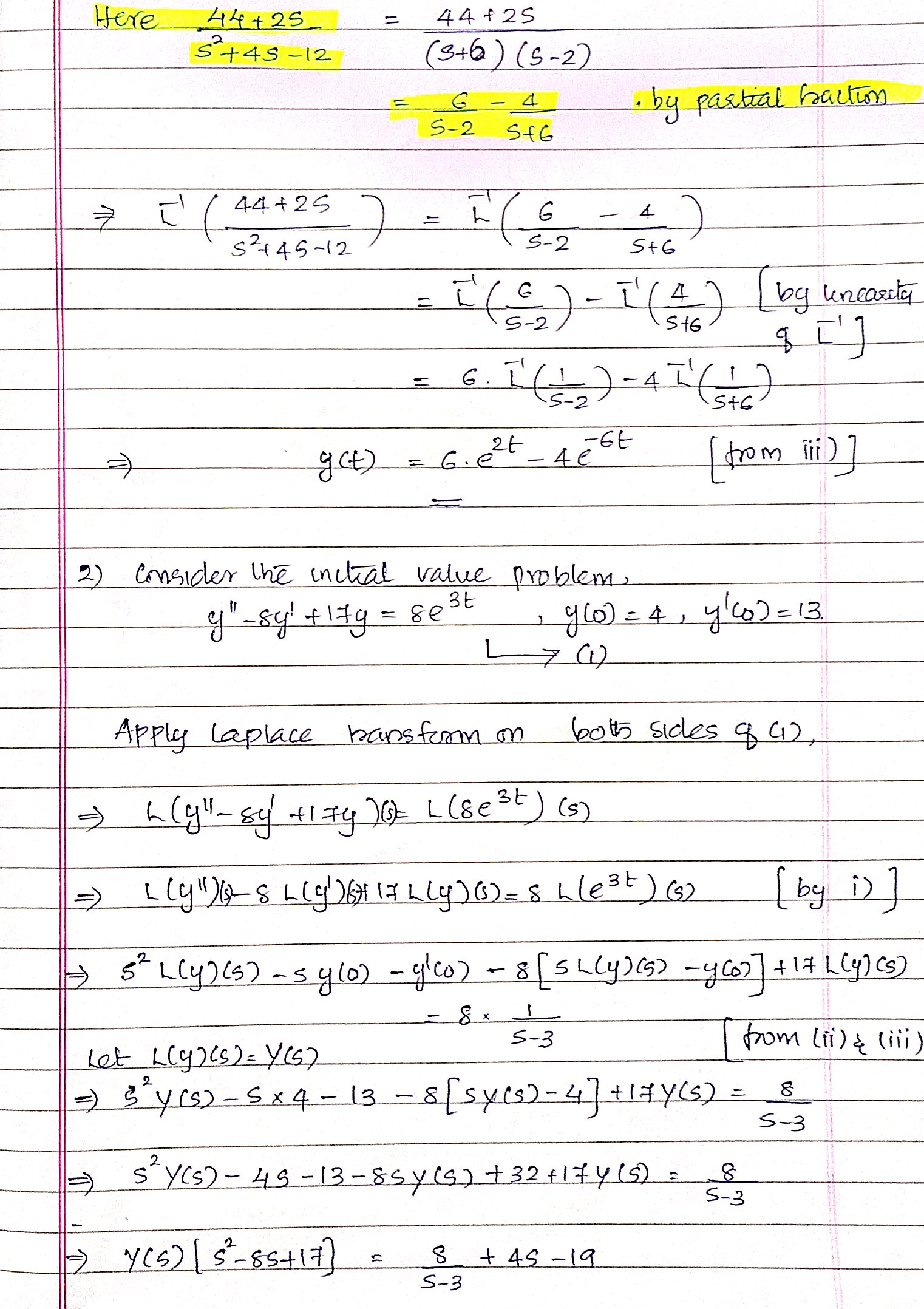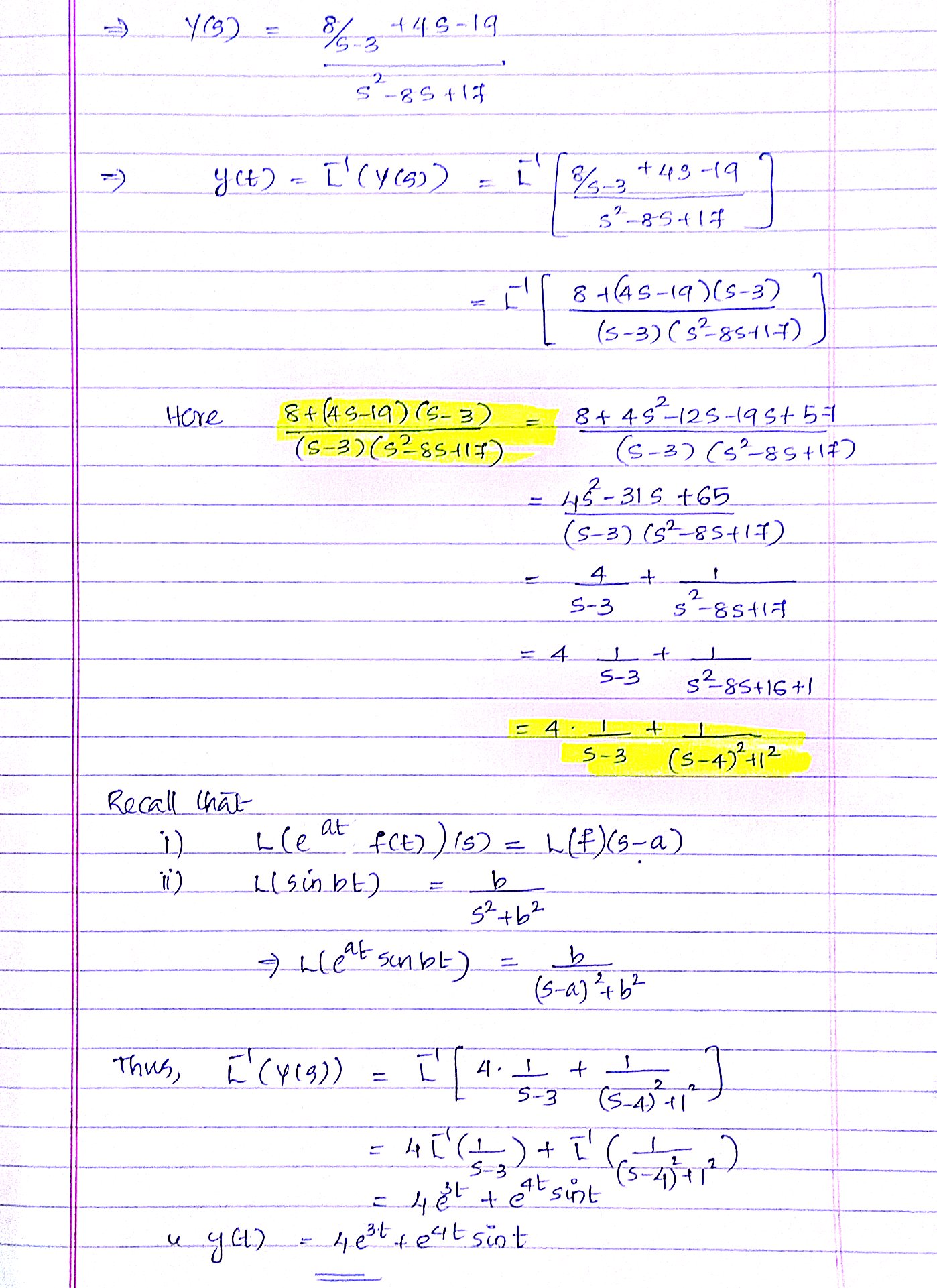Similar Homework Help Questions
• ### Solve the initial value problem below using the method of Laplace transforms. 2ty" - 5ty' +...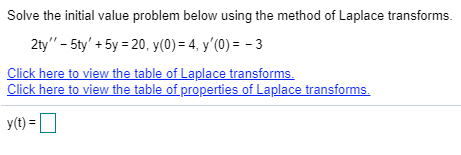Solve the initial value problem below using the method of Laplace transforms. 2ty" - 5ty' + 5y = 20, y(0) = 4, y'0) = -3 Click here to view the table of Laplace transforms. Click here to view the table of properties of Laplace transforms. y(t) =

• ### Solve the initial value problem below using the method of Laplace transforms. y"' + y' -...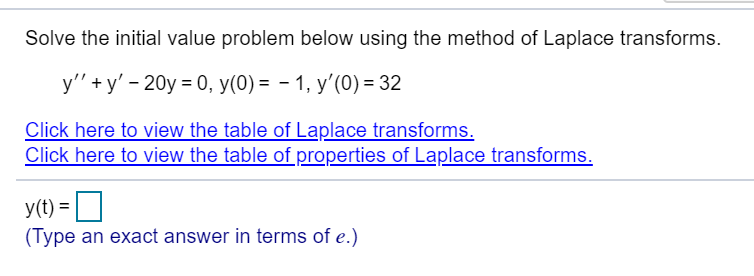Solve the initial value problem below using the method of Laplace transforms. y"' + y' - 20y = 0, y(0) = -1, y'(0) = 32 Click here to view the table of Laplace transforms. Click here to view the table of properties of Laplace transforms. y(t) = (Type an exact answer in terms of e.)

• ### Solve the initial value problem below using the method of Laplace transforms. y" - 2y' -...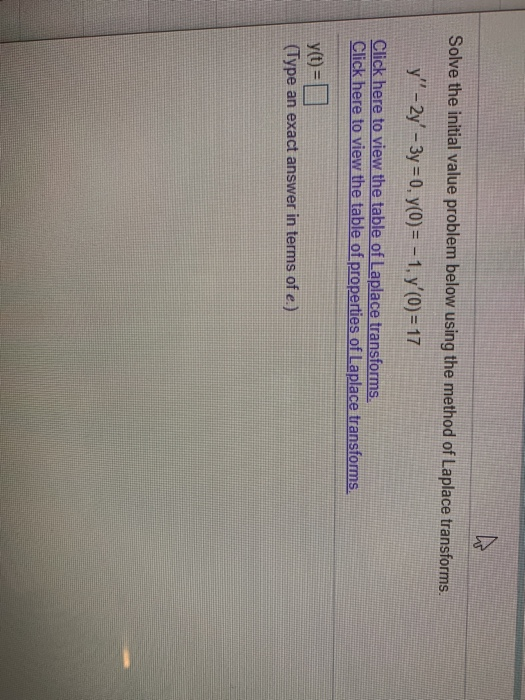Solve the initial value problem below using the method of Laplace transforms. y" - 2y' - 3y = 0, y(0) = -1, y' (O) = 17 Click here to view the table of Laplace transforms. Click here to view the table of properties of Laplace transforms y(t) = 1 (Type an exact answer in terms of e.)

• ### Solve the initial value problem below using the method of Laplace transforms. y'' - 12y' +45y...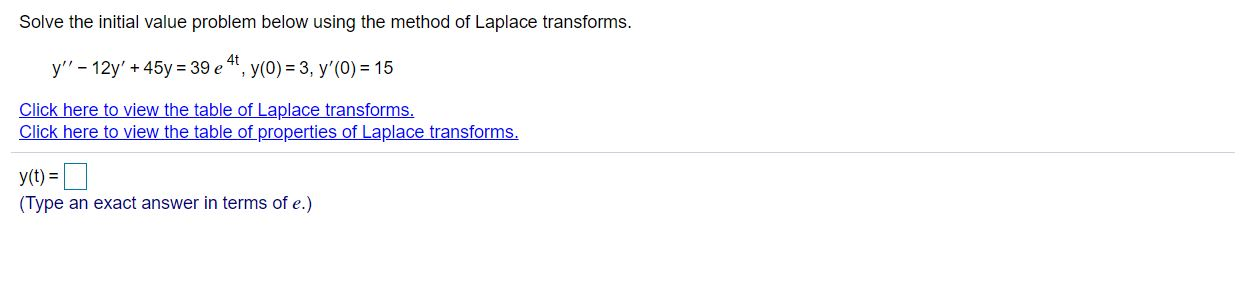Solve the initial value problem below using the method of Laplace transforms. y'' - 12y' +45y = 39 e 4t, y(0) = 3, y'(0) = 15 Click here to view the table of Laplace transforms. Click here to view the table of properties of Laplace transforms. y(t) = (Type an exact answer in terms of e.)

• ### Solve for Y(s), the Laplace transform of the solution y(t) to the initial value problem below...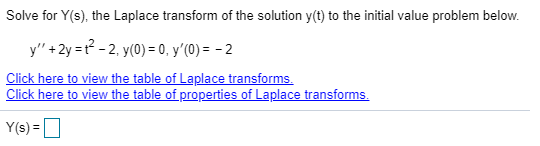Solve for Y(s), the Laplace transform of the solution y(t) to the initial value problem below y+2yt22, y(0) = 0, y'(0) = - 2 Click here to view the table of Laplace transforms Click here to view the table of properties of Laplace transforms Y(s)= Solve for Y(s), the Laplace transform of the solution y(t) to the initial value problem below y+2yt22, y(0) = 0, y'(0) = - 2 Click here to view the table of Laplace transforms Click here...

• ### Solve for Y(s), the Laplace transform of the solution y(t) to the initial value problem below....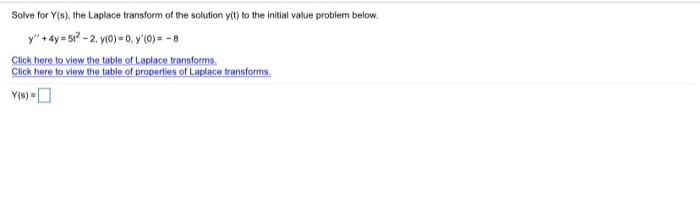Solve for Y(s), the Laplace transform of the solution y(t) to the initial value problem below. y" + 4y = 512 - 2. y(0)=0, 7(0) = -8 Click here to view the table of Laplace transforms Click here to view the table of properties of Laplace transforms. Solve for Y(s), the Laplace transform of the solution y(t) to the initial value problem below. y" + 4y = 5t2 - 2. y(0) = 0, y'(O) = - 8 Click here to...

• ### Solve the initial value problem below using the method of Laplace transforms. 4ty'' - 6ty' +...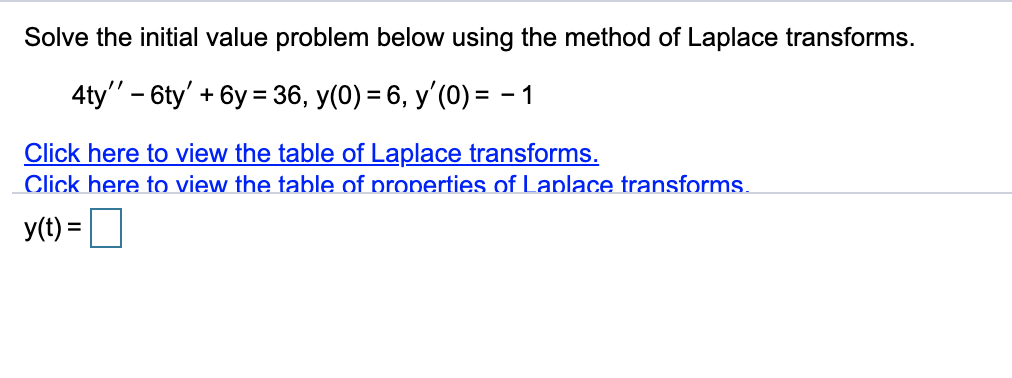Solve the initial value problem below using the method of Laplace transforms. 4ty'' - 6ty' + 6y = 36, y(0) = 6, y'(0) = -1 Click here to view the table of Laplace transforms. Click here to view the table of properties of Laplace transforms. y(t) =

• ### Solve for Y(s), the Laplace transform of the solution y(t) to the initial value problem below....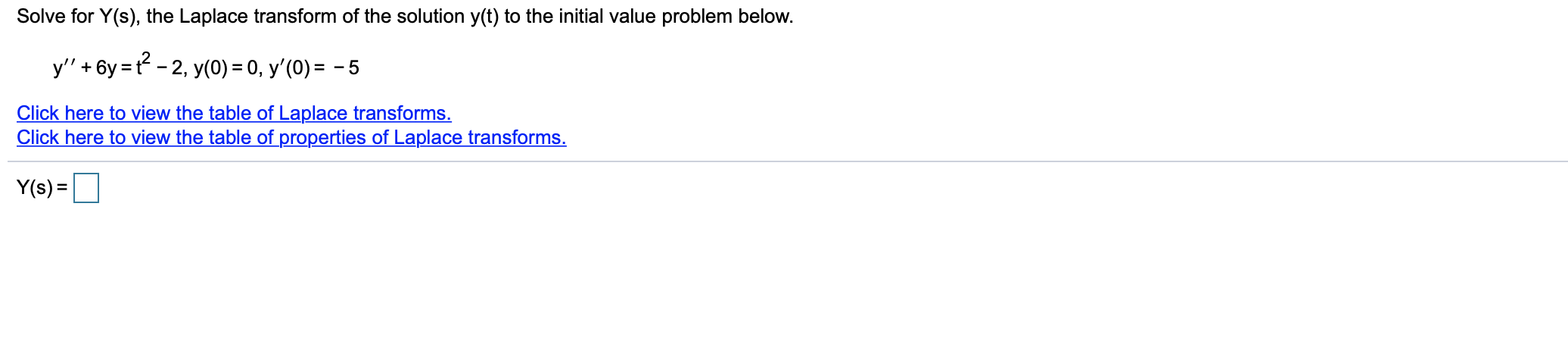Solve for Y(s), the Laplace transform of the solution y(t) to the initial value problem below. y'' +6y=t? - 2, y(0) = 0, y'(0) = -5 Click here to view the table of Laplace transforms. Click here to view the table of properties of Laplace transforms. Y(s)=

• ### Solve for Y(s), the Laplace transform of the solution y(t) to the initial value problem below....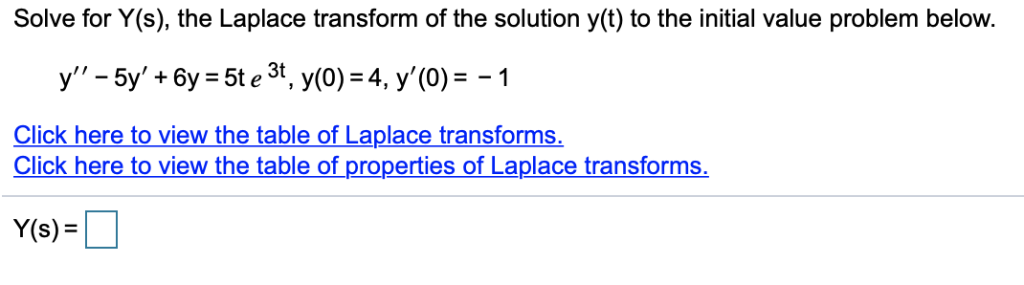Solve for Y(s), the Laplace transform of the solution y(t) to the initial value problem below. Click here to view the table of Laplace transforms. Click here to view the table of properties of Laplace transforms Y(s)

• ### Solve for Y(s), the Laplace transform of the solution y(t) to the initial value problem below....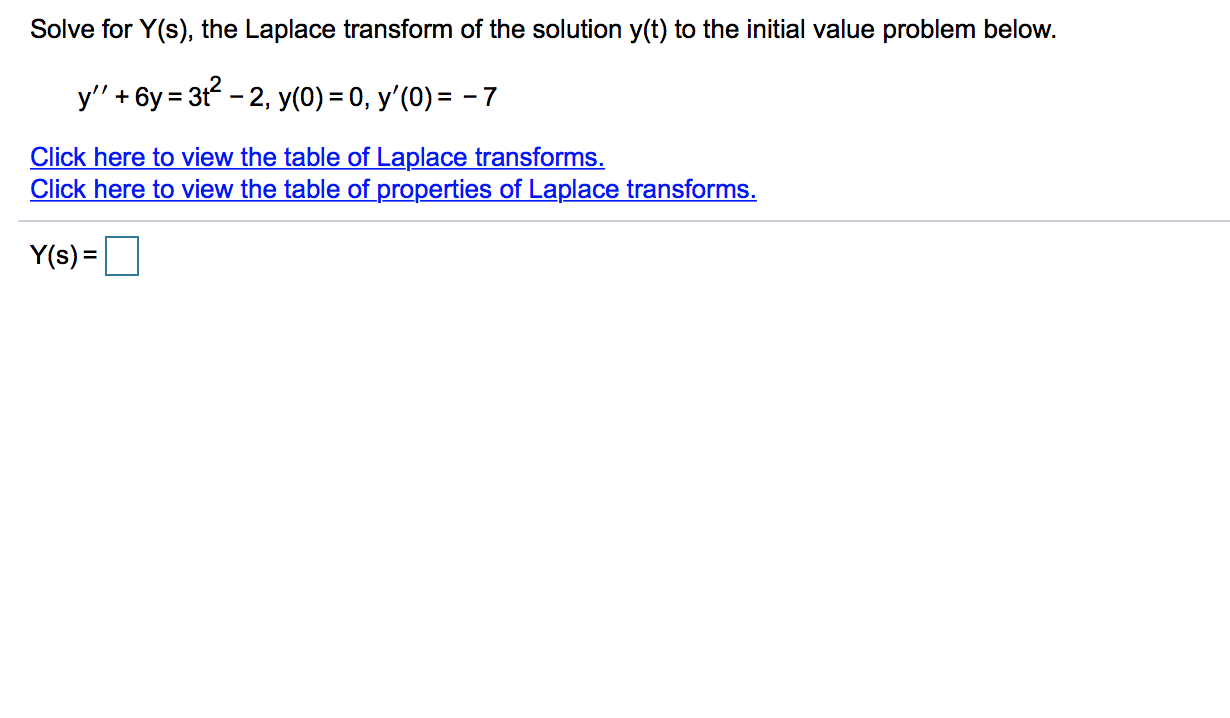Solve for Y(s), the Laplace transform of the solution y(t) to the initial value problem below. y' + 6y = 312 - 2, y(0) = 0, y'(0) = - 7 Click here to view the table of Laplace transforms. Click here to view the table of properties of Laplace transforms. Y(s)-

Free Homework App